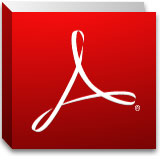You are hereCourses / Mathematics / Advanced Math S2 (#462)

Textbook:

This course is an online course through mathXLforschool.com. It is based on Prentice Hall Algebra 2 and Trigonometry (New York Edition). Copyright 2013 by Pearson Education.

Course Description:

You will study the topics from the first six chapters of the textbook. A list of the major topics is given below:

Chapter 7
Exponential and Logarithmic Functions
Exploring exponential growth and decay
Graph exponential functions
Evaluate powers of e
Convert between exponential form and logarithmic form
Evaluate logarithms

Chapter 8
Rational Functions
Use properties of logarithms
Change of base formula
Solve logarithmic equations
Natural logarithms

Chapter 9
Sequences and Series
Recognize and use inverse variation
Reciprocal functions
Rational expressions and functions
Complex fractions
Arithmetic sequences
Geometric sequences
Sums of arithmetic series
Sums of finite geometric series

Chapter 10
Graph and identify conic sections
Vertex, focus, and directrix of a parabola
Circles, Ellipse, and Hyperbola

Midcourse Exam on Chapters 7 – 10

Chapter 11
Probability and Statistics
Combinations and Permutations
Theoretical vs empirical methods
Factorials

Chapter 12
Matrices
Multiply matricies
Scalar products
Inverse of a matrix

Chapter 13
Periodic Functions and Trigonometry
Cycles and Periods
Coterminal angles
Sine, Cosine and Tangent
Arc Length
Sine, Cosine and tangent equations and graphs
Phase shifts and translations

Chapter 14
Trigonometric Identities and Equations
Unit circle
Inverse functions
Express trigonometric functions in terms of another
The six trigonometric functions of an angle
Law of Sines
Law of Cosines
Angle identities
Double-angle identity

Final Exam on Chapters 11 – 14

## Important notice regarding our English course offering:

All English courses (listed) are temporarily suspended until further notice: English I S1 (#311), English I S2 (#312), English II S1 (#321), English II S2 (#322), English III S1 (#331), English III S2 (#332), English IV S1 (#341), and English IV S2 (#342).

## Notice to Prospective StudentsStudents must have a valid e-mail address, physical mailing address and access to any free PDF file viewer, like Adobe Reader, for filling out course materials online and printing course materials at home.